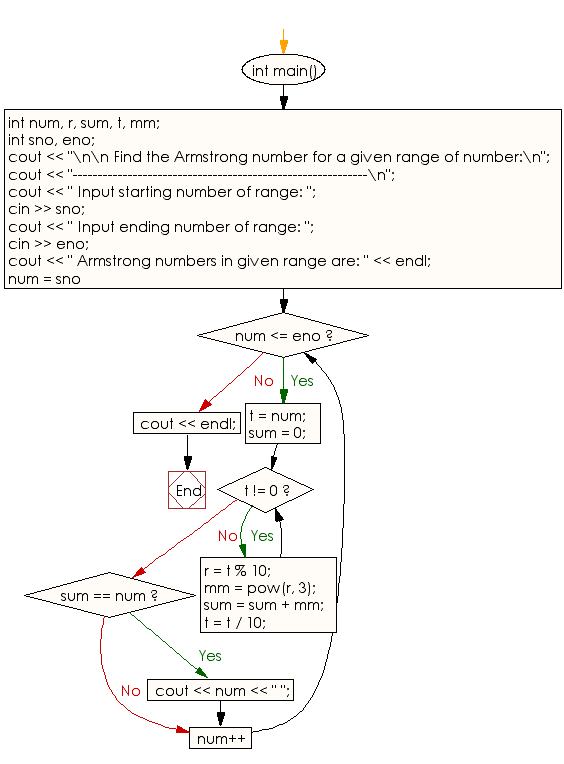﻿ C++ : Find the Armstrong number for a given range of number

# C++ Exercises: Find the Armstrong number for a given range of number

## C++ Numbers: Exercise-44 with Solution

Write a C++ program to find the Armstrong number for a given range of numbers.

/*When the sum of the cube of the individual digits of a number
is equal to that number, the number is called Armstrong number. For example 153.
Sum of its divisor is 13 + 53;+ 33; = 1+125+27 = 153*/

Sample Solution:

C++ Code:

``````#include <iostream>
#include <math.h>
using namespace std;

int main()
{
int num, r, sum, t, mm;
int sno, eno;
cout << "\n\n Find the Armstrong number for a given range of number:\n";
cout << "-----------------------------------------------------------\n";
cout << " Input starting number of range: ";
cin >> sno;
cout << " Input ending number of range: ";
cin >> eno;
cout << " Armstrong numbers in given range are: " << endl;
for (num = sno; num <= eno; num++)
{
t = num;
sum = 0;
while (t != 0)
{
r = t % 10;
mm = pow(r, 3);
sum = sum + mm;
t = t / 10;
}
if (sum == num)
cout << num << " ";
}
cout << endl;
}
``````

Sample Output:

``` Find the Armstrong number for a given range of number:
-----------------------------------------------------------
Input starting number of range: 25
Input ending number of range: 200
Armstrong numbers in given range are:
153
```

Flowchart:C++ Code Editor: